萬字詳解什麼是生成對抗網路GAN

一.GAN簡介

1.GAN背景知識

Ian Goodfellow 因提出了生成對抗網路（GANs，Generative Adversarial Networks）而聞名， GAN最早由Ian Goodfellow於2014年提出，以其優越的效能，在不到兩年時間裡，迅速成為一大研究熱點。他也被譽為“GANs之父”，甚至被推舉為人工智慧領域的頂級專家。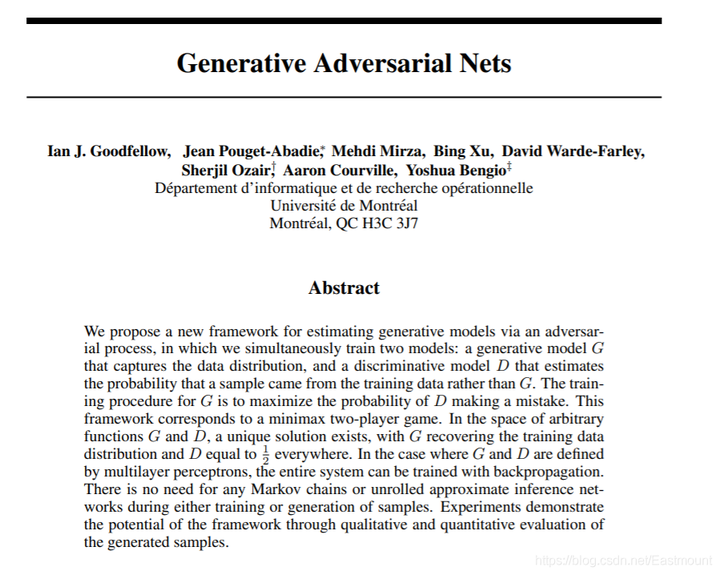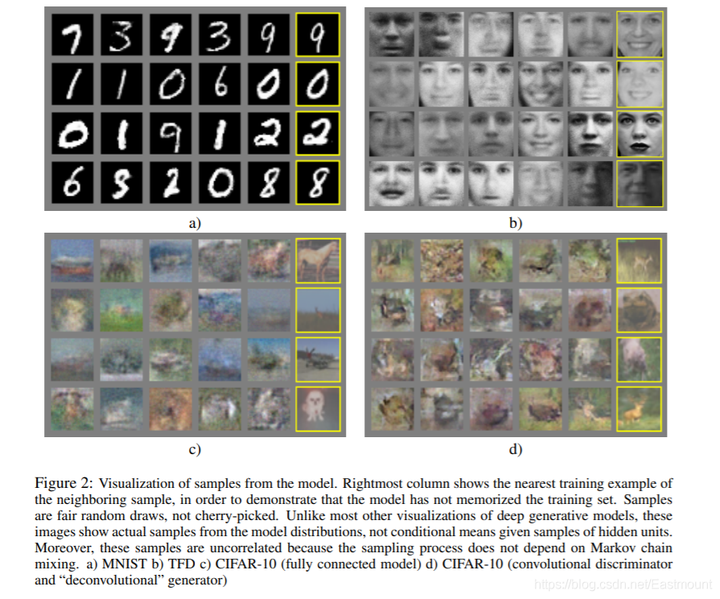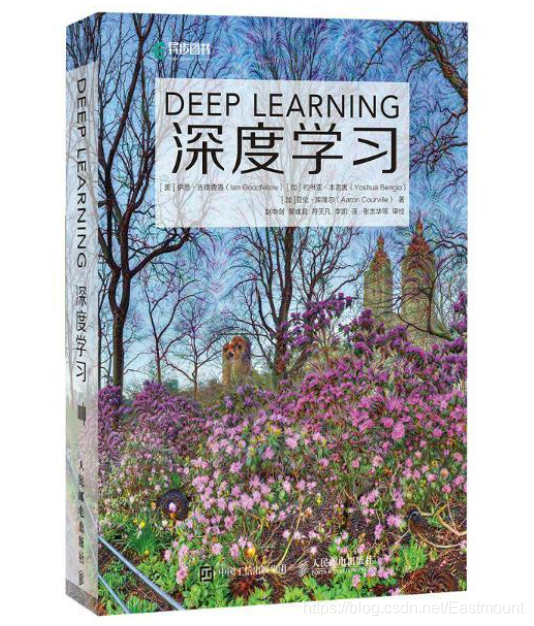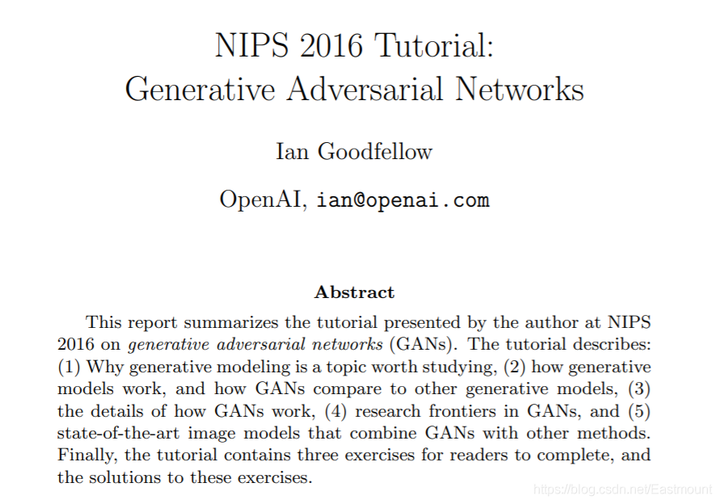Yann LeCun稱GAN為“過去十年機器學習界最有趣的idea”。GAN在github上的火熱程度如下圖所示，呈指數增漲，出現各種變形。當然，其中也存在很多比較水的文章，推薦大家儘量學習比較經典的模型。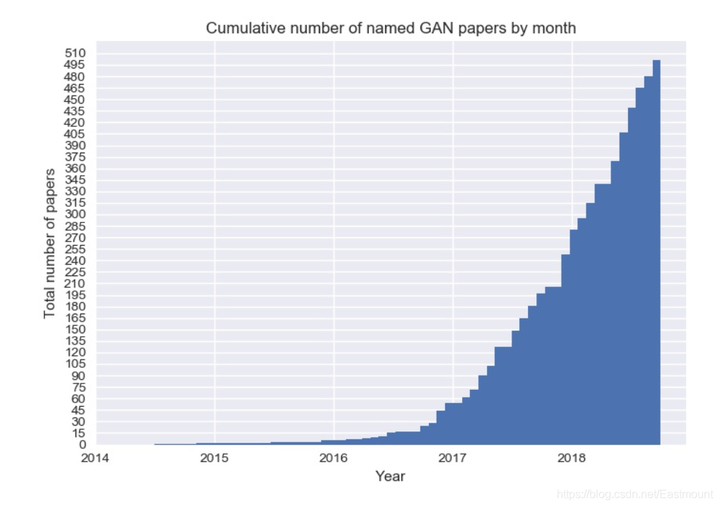2.GAN原理解析

• Generative：生成式模型
• Networks：網路（不一定是深度學習）

GANs是一類生成模型，從字面意思不難猜到它會涉及兩個“對手”，一個稱為Generator（生成者），一個稱為Discriminator（判別者）。Goodfellow最初arxiv上掛出的GAN tutorial文章中將它們分別比喻為偽造者（Generator）和警察（Discriminator）。偽造者總想著製造出能夠以假亂真的鈔票，而警察則試圖用更先進的技術甄別真假。兩者在博弈過程中不斷升級自己的技術。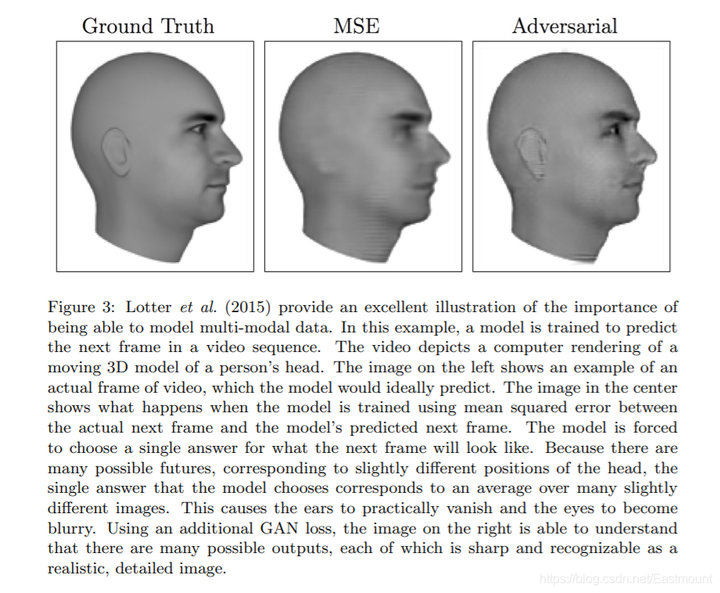• bicubic：第二張圖是bicubic方法恢復的影象。經過壓縮再拉伸還原影象，通過插值運算實現，但其影象會變得模糊。
• SRResNet：第三張影象是通過SRResNet實現的恢復，比如先壓縮影象再用MSE和神經網路學習和真實值的差別，再進行恢復。（SRResNet is a neural network trained with mean squared error）
• SRGAN：第四張圖是通過SRGAN實現的，其恢復效果更優。SRGAN是在GAN基礎上的改進，它能夠理解有多個正確的答案，而不是在許多答案中給出一個最佳輸出。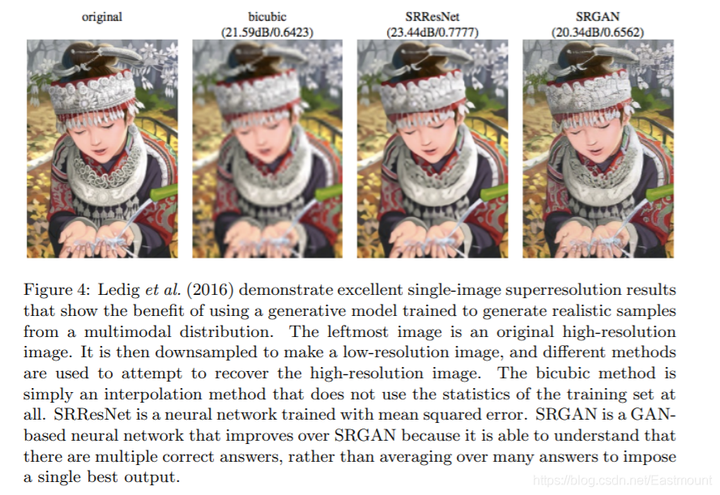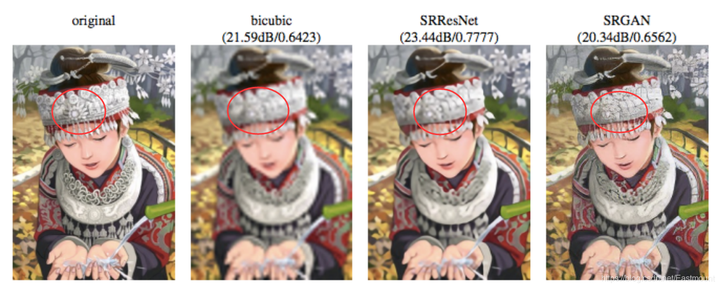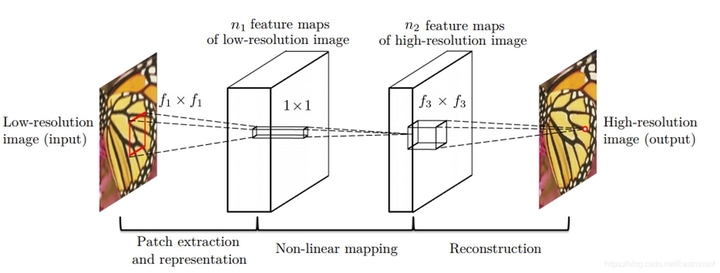(1) 使用了一個卷積神經網路來進行超分，端到端的學習低解析度與超解析度之間的對映。
(2) 將提出的神經網路模型與傳統的稀疏編碼方法之間建立聯絡，這種聯絡還指導用來設計神經網路模型。
(3) 實驗結果表明深度學習方法可以用於超分中，可以獲得較好的質量和較快的速度。

3.GAN經典案例

GNN究竟能做什麼呢？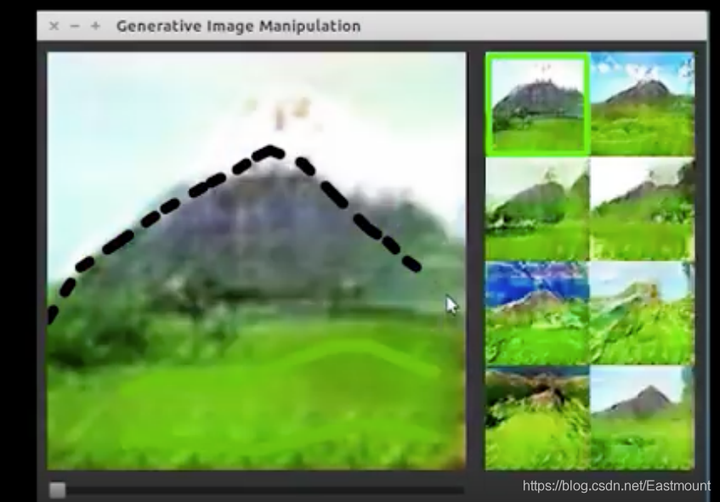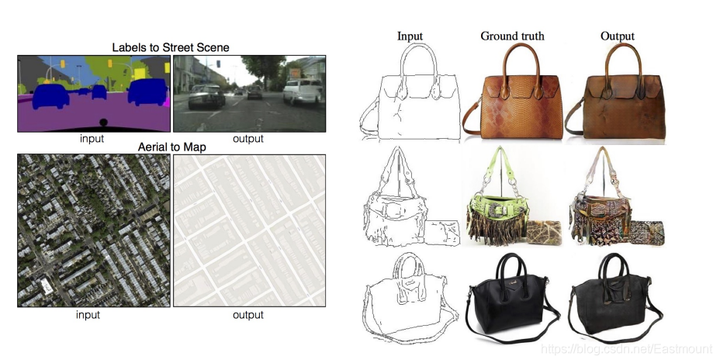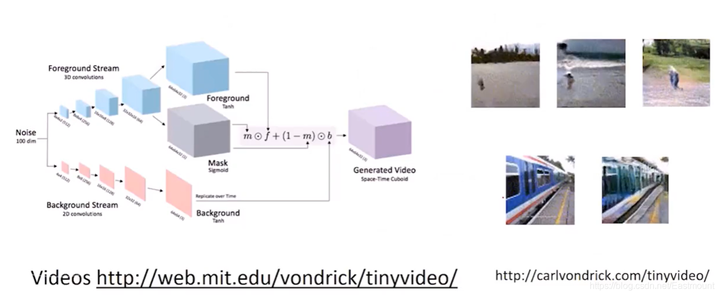Wu等在NIPS 2016中通過GAN實現了用噪聲去生成一張3D椅子模型。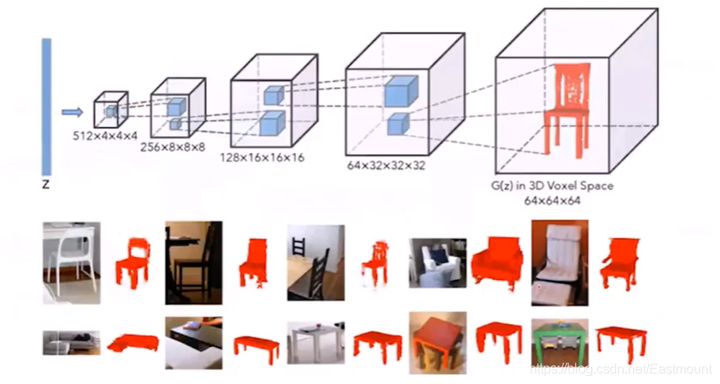starGAN轉移從RaFD資料集中學到的知識，在CelebA資料集上的多域影象轉換結果。第一和第六列顯示輸入影象，其餘列是由starGAN生成的影象。請注意，這些影象是由一個單一的生成器網路生成的，而憤怒、快樂和恐懼等面部表情標籤都來自RaFD，而不是CelebA。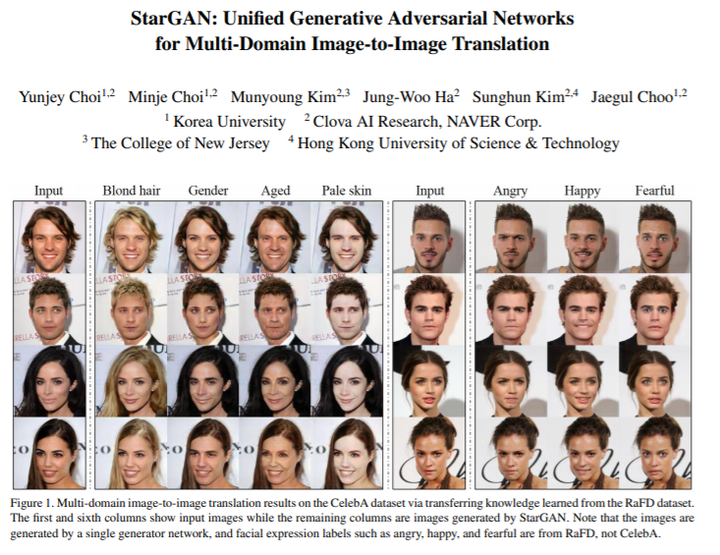二.GAN預備知識

1.什麼是神經網路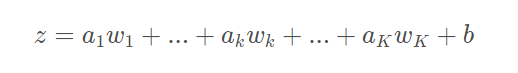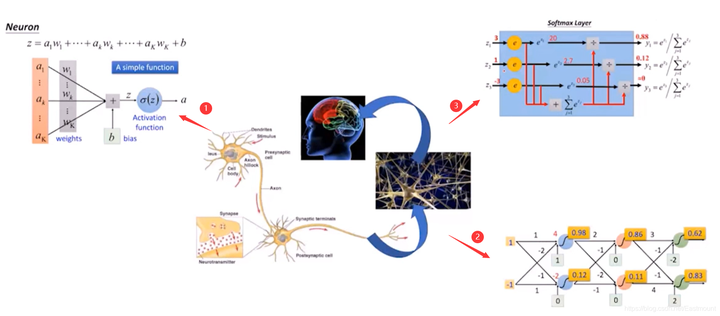• 神經網路常見層
全連線層、啟用層、BN層、Dropout層、卷積層、池化層、迴圈層、Embedding層、Merege層等
• 網路配置
損失函式、優化器、啟用函式、效能評估、初始化方法、正則項等
• 網路訓練流程
預訓練模型、訓練流程、資料預處理（歸一化、Embedding）、資料增強（圖片翻轉旋轉曝光生成海量樣本）等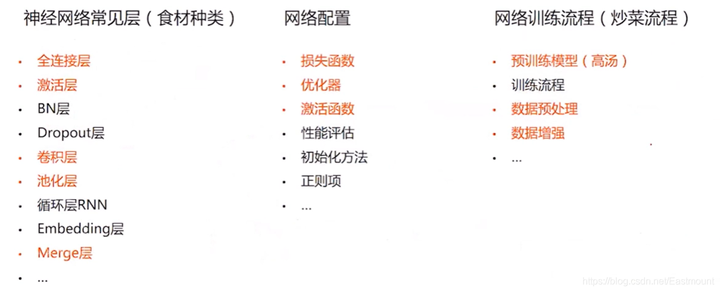2.全連線層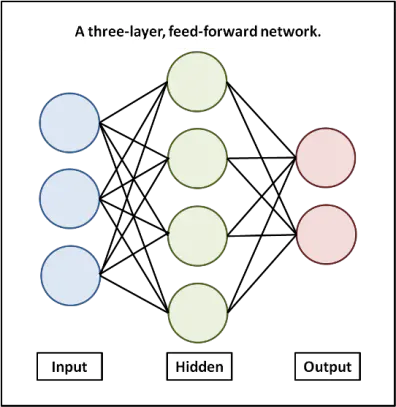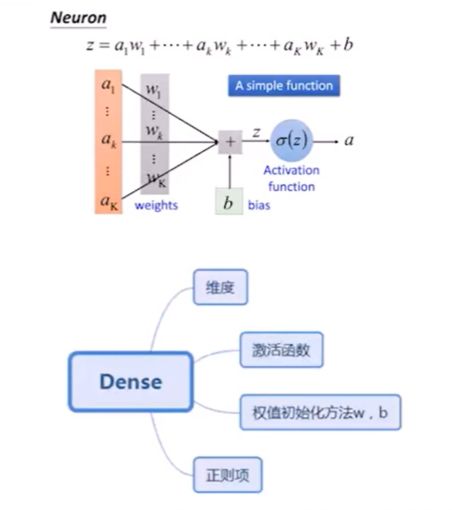3.啟用函式

• 對於隱藏層，我們可以使用relu、tanh、softplus等非線性關係；
• 對於分類問題，我們可以使用sigmoid（值越小越接近於0，值越大越接近於1）、softmax函式，對每個類求概率，最後以最大的概率作為結果；
• 對於迴歸問題，可以使用線性函式（linear function）來實驗。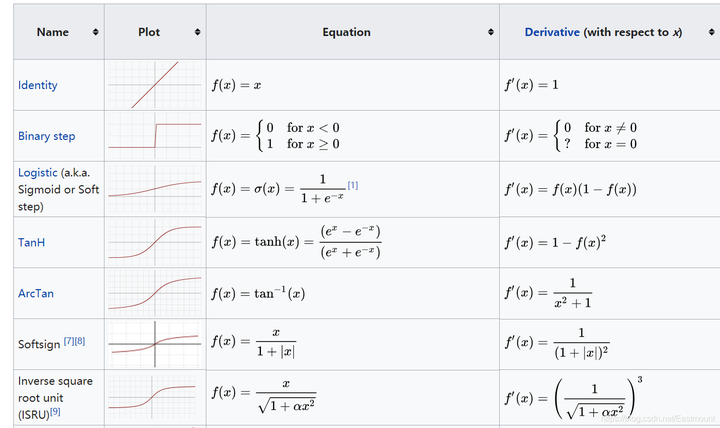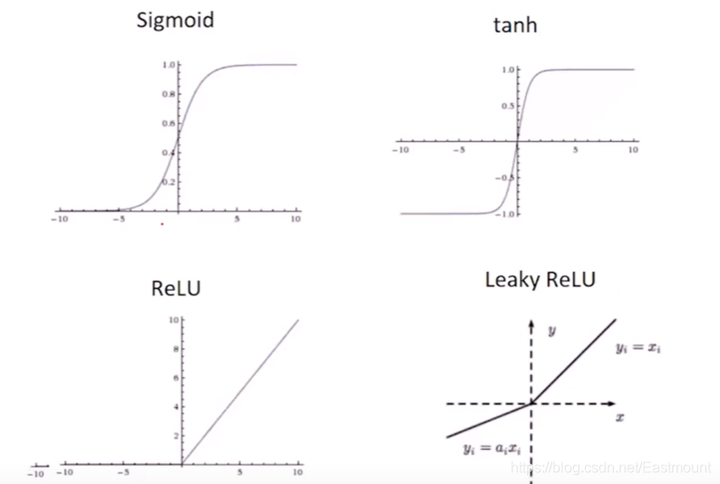4.反向傳播

BP神經網路是非常經典的網路，這裡通過知乎EdisonGzq大佬的兩張圖來解釋神經網路的反向傳播。對於一個神經元而言，就是計算最後的誤差傳回來對每個權重的影響，即計算每層反向傳遞的梯度變化。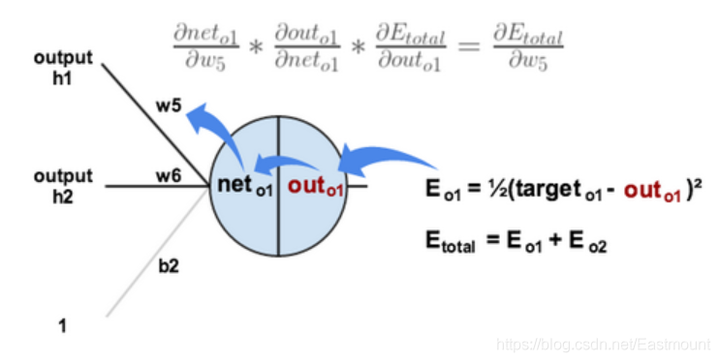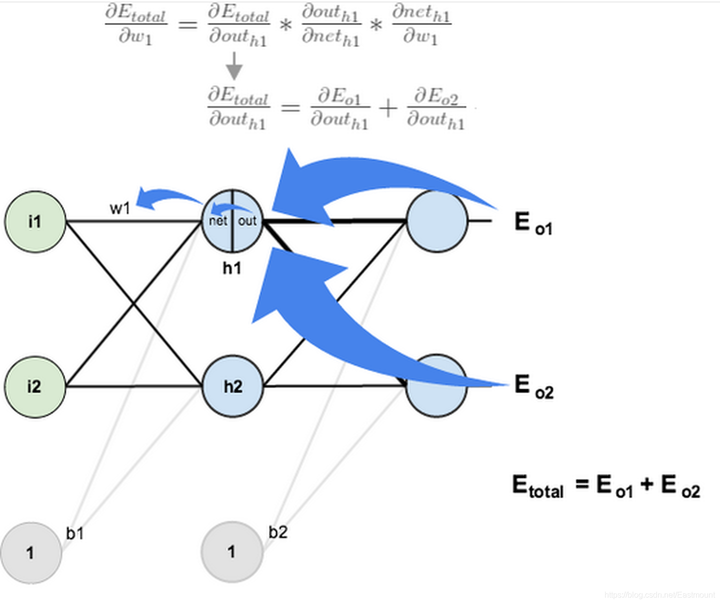5.優化器選擇

• 一種優化器是跟著梯度走，每次只觀察自己的梯度，它不帶重量
• 一種優化器是帶重量的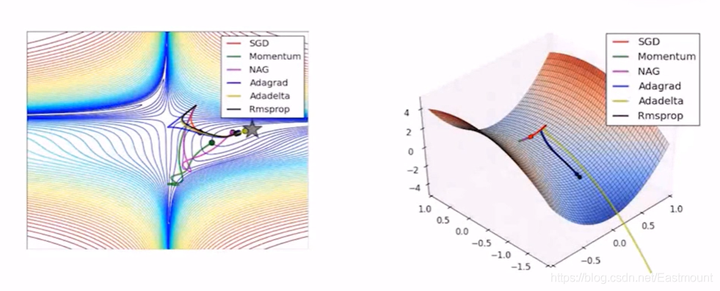• class tf.train.MomentumOptimizer
• class tf.train.FtrlOptimizer
• class tf.train.RMSPropOptimizer

• MomentumOptimizer
• RMSPropOptimizer6.卷積層

• 圖片引數太多，比如1000*1000的圖片，加一個隱藏層，隱藏層節點同輸入維數，全連線的引數是10^12，根本訓練不過來這麼多引數。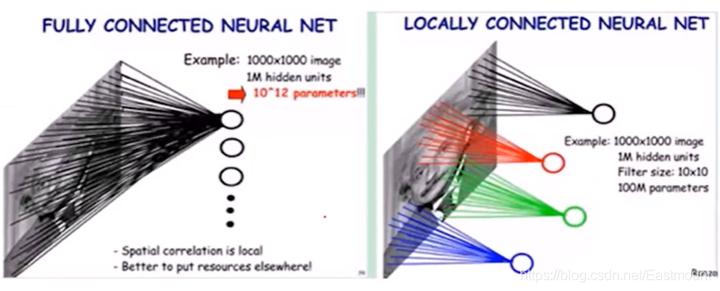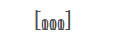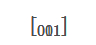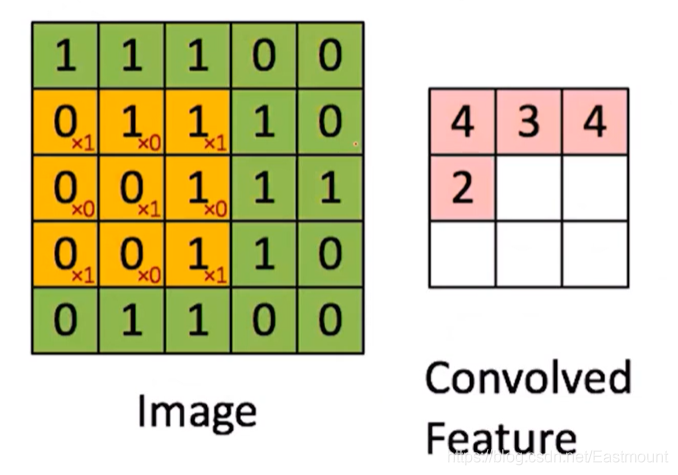• 卷積核數目
• 卷積核大小：如上面3x3卷積核
• 卷積核數目
• 卷積核步長：上面的步長是1，同樣可以調格
• 啟用函式
• 是否使用偏置
• 學習率
• 初始化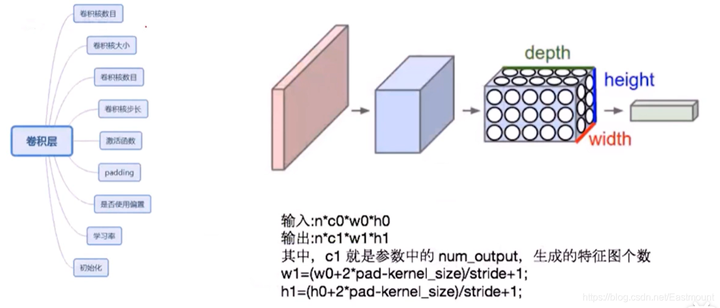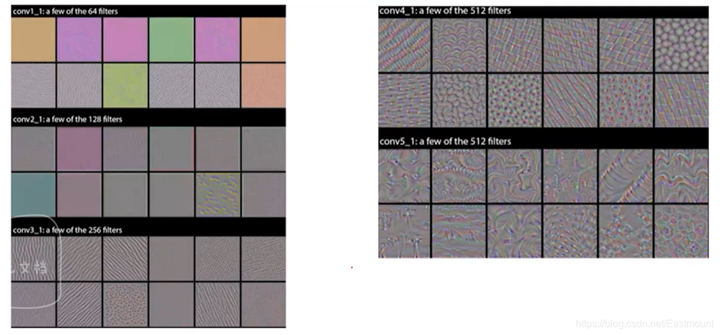7.池化層

• 使特徵圖變小，簡化網路；特徵壓縮，提取主要特徵

• 最大池化：比如從左上角紅色區域中選擇最大的6，接著是8、3、4
• 平均池化：選擇平均值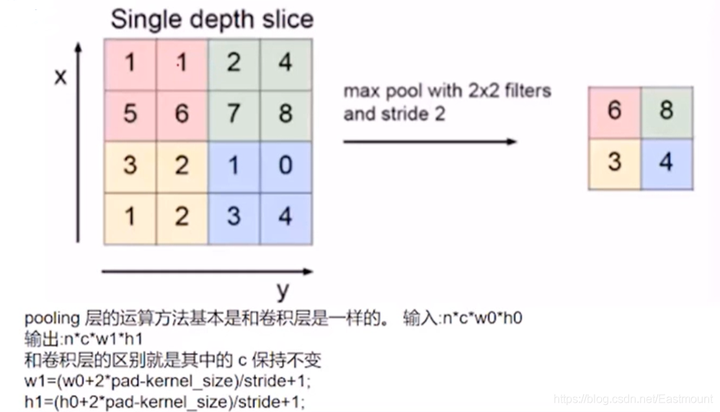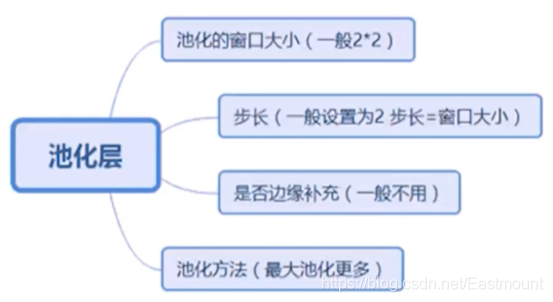8.影象問題基本思路

• 輸入層
如NLP句子、句對，影象的畫素矩陣，語音的音訊資訊
• 表示成
DNN：全連線+非線性（特徵非線性融合）
CNN：Conv1d、Conv2d、Pooling
RNN：LSTM、GRU（選擇記憶性）
• 應用層
分類、迴歸、序列預測、匹配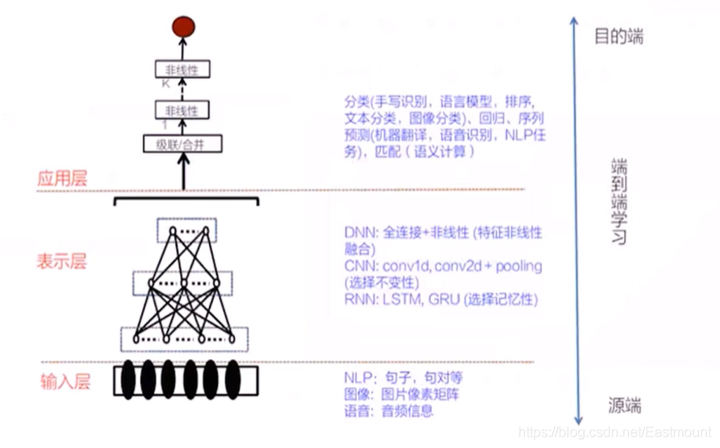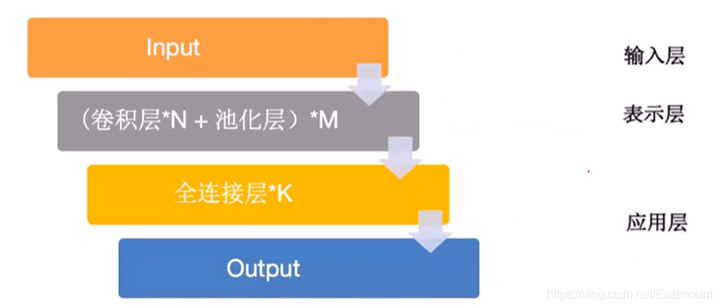三.GAN網路實戰分析

• Generative：生成式模型
• Networks：網路

1.GAN模型解析

• 最開始在圖(a)中我們生成綠線，即生成樣本的概率分佈，黑色的散點是真實樣本的概率分佈，這條藍線是一個判決器，判斷什麼時候應該是真的或假的。
• 我們第一件要做的事是把判決器判斷準，如圖(b)中藍線，假設在0.5的位置下降，之前的認為是真實樣本，之後的認為是假的樣本。
• 最終輸出圖(d)，如果你真實的樣本和生成的樣本完全一致，分佈完全一致，判決器就傻了，無法繼續判斷。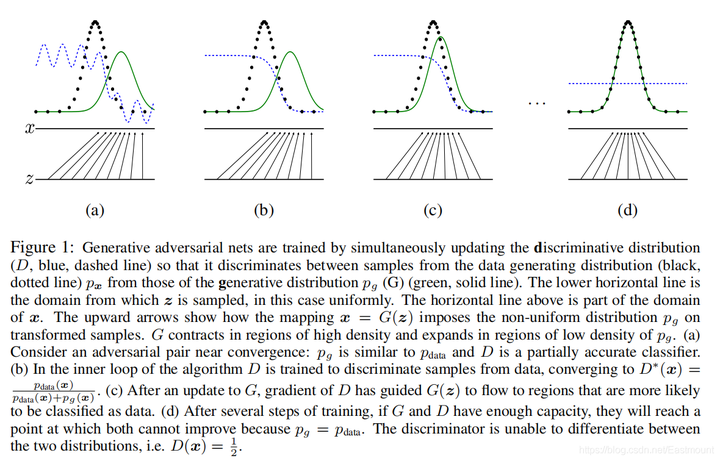• 生成器：學習真實樣本以假亂真
• 判別器：小孩通過學習成驗鈔機的水平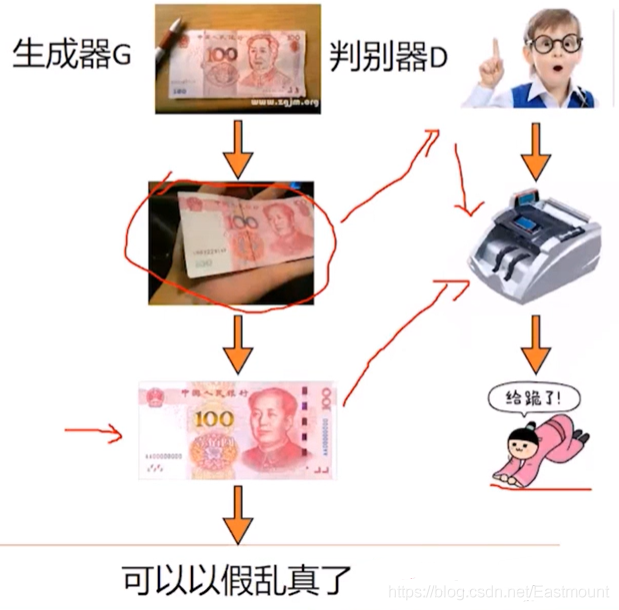GAN的整體思路是一個生成器，一個判別器，並且GoodFellow論文證明了GAN全域性最小點的充分必要條件是：生成器的概率分佈和真實值的概率分佈是一致的時候。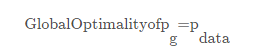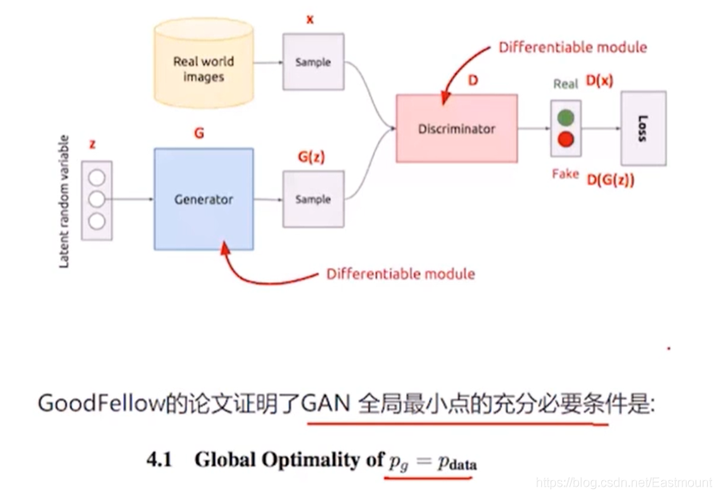• 目標函式如何設定？
• 如何生成圖片？
• G生成器和D判決器應該如何設定？
• 如何進行訓練？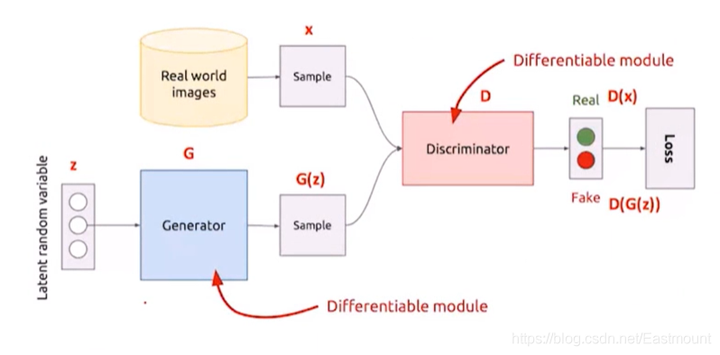(1) 目標函式

• max()式子是第一步，表示把生成器G固定，讓判別器儘量區分真實樣本和假樣本，即希望生成器不動的情況下，判別器能將真實的樣本和生成的樣本區分開。
• min()式子是第二步，即整個式子。判別器D固定，通過調整生成器，希望判別器出現失誤，儘可能不要讓它區分開。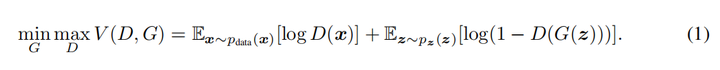• 式子由兩項構成，x表示真實圖片，z表示輸入G網路的噪聲，而G(z)表示G網路生成的圖片。
• D(x)表示D網路判斷真實圖片是否真實的概率（因為x就是真實的，所以對於D來說，這個值越接近1越好）。
• D(G(z))是D網路判斷G生成的圖片是否真實的概率。
• G的目的：G應該希望自己生成的的圖片越接近真實越好。
• D的目的：D的能力越強，D(x)應該越大，D(G(x))應該越小，這時V(D,G)會變大，因此式子對於D來說是求最大（max_D）。
• trick：為了前期加快訓練，生成器的訓練可以把log(1-D(G(z)))換成-log(D(G(z)))損失函式。

• 最外層是一個for迴圈，接著是k次for迴圈，中間迭代的是判決器。
• k次for迴圈結束之後，再迭代生成器。
• 最後結束迴圈。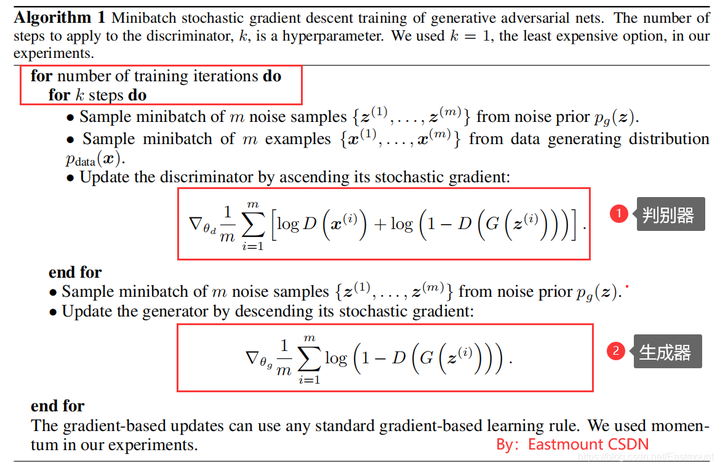(2) GAN圖片生成

• 第一步（左圖）：希望判決器儘可能地分開真實資料和我生成的資料。那麼，怎麼實現呢？我的真實資料就是input1（Real World images），我生成的資料是input2（Generator）。input1的正常輸出是1，input2的正常輸出是0，對於一個判決器（Discriminator）而言，我希望它判決好，首先把生成器固定住（虛線T），然後生成一批樣本和真實資料混合給判決器去判斷。此時，經過訓練的判決器變強，即固定生成器且訓練判決器。
• 第二步（右圖）：固定住判決器（虛線T），我想辦法去混淆它，剛才經過訓練的判決器很厲害，此時我們想辦法調整生成器，從而混淆判別器，即通過固定判決器並調整生成器，使得最後的輸出output讓生成的資料也輸出1（第一步為0）。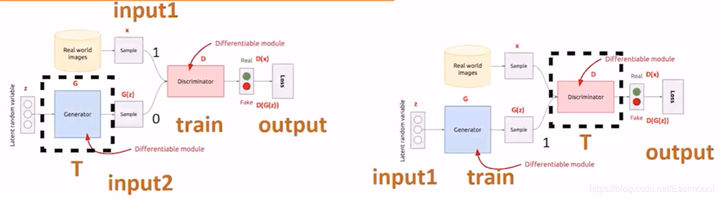GAN的核心就是這些，再簡單總結下，即：

• 步驟1是在生成器固定的時候，我讓它產生一批樣本，然後讓判決器正確區分真實樣本和生成樣本。（生成器標籤0、真實樣本標籤1）
• 步驟2是固定判決器，通過調整生成器去儘可能的瞞混判決器，所以實際上此時訓練的是生成器。（生成器的標籤需要讓判決器識別為1，即真實樣本）

for 迭代 in range(迭代總數):
for batch in range(batch_size):
新batch = input1的batch + input2的batch (batch加倍)
for 輪數 in range(判別器中輪數):
步驟一 訓練D
步驟二 訓練G

2.生成手寫數字demo分析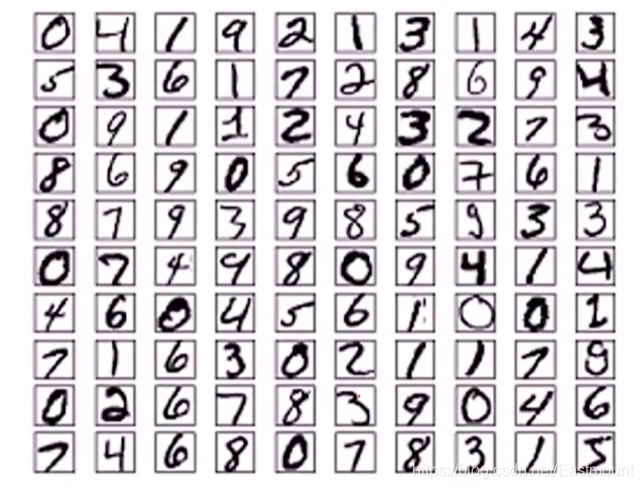(1) 生成器G

• 全連線層：輸入100維，輸出1024維
• 全連線層：128x7x7表示圖片128通道，大小7x7
• BatchNormalization：如果不加它DCGAN程式會奔潰
• UpSampling2D：對卷積結果進行上取樣從而將特徵圖放大 14x14
• Conv2D：卷積操作畫素尺度不變（same）
• UpSampling2D：生成28x28
• Conv2D：卷積操作
• Activation：啟用函式tanh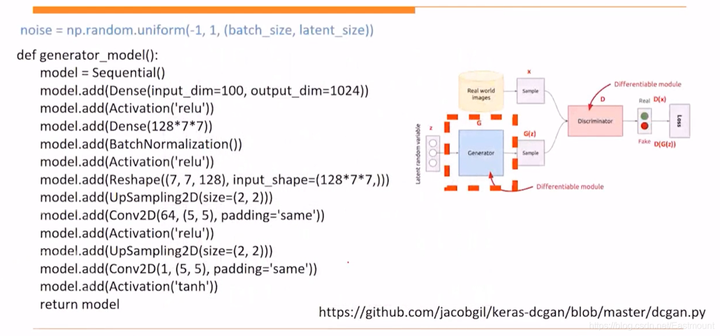(2) 判別器D

• Conv2D：卷積層
• MaxPooling2D：池化層
• Conv2D：卷積層
• MaxPooling2D：池化層
• Flatten：拉直一維
• Dense：全連線層
• Activation：sigmoid二分類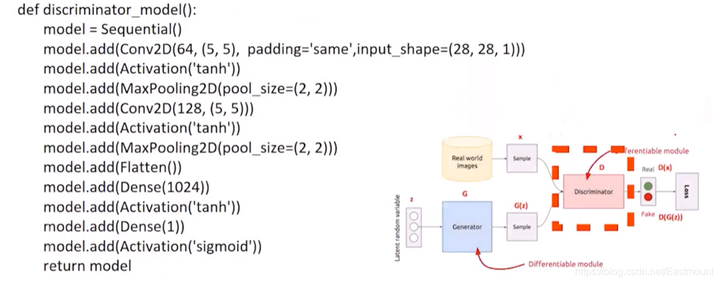(3) 輔助函式

• d.trainable=False：判決器D固定

combine_images函式實現合併影象的操作。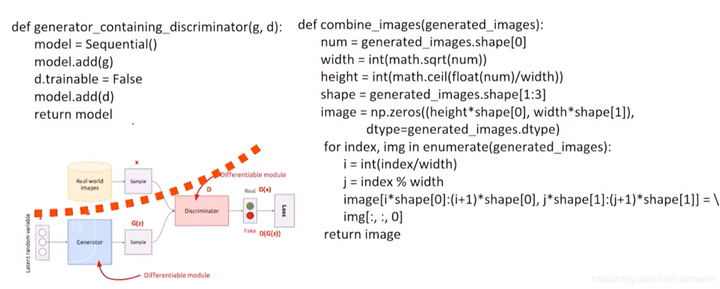(4) GAN圖片生成訓練
GAN核心流程包括：

• d = discriminator_model：定義判別器D
• g = generator_model：定義生成器G
• generator_containing_discriminator：固定D調整G
• SGD、compile：定義引數、學習率
• for epoch in range、for index in rangeBATCH
• X = np.concatenate：影象資料和生成資料混合
• y =  x BATCH_SIZE +  x BTCH_SIZE：輸出label
• d_loss = d.train_on_batch(X,y)：訓練D判別器（步驟一）
• d.trainable = False：固定D
• g_loss = d_on_g.train_on_batch(noise, xBATCH_SIZE)：訓練G生成器（步驟二），混淆
• d.trainable = True：開啟D重複操作
• 儲存引數和模型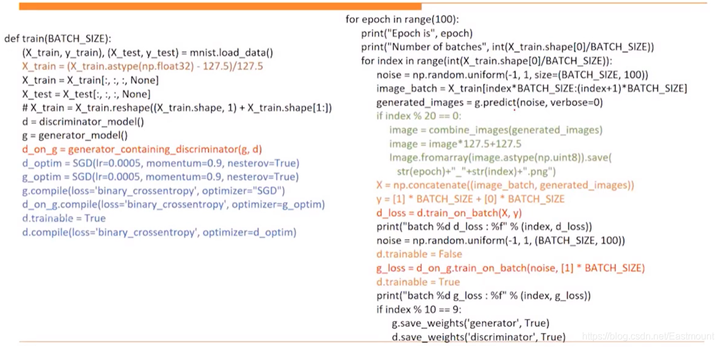(5) 生成

• g = generator_model：定義生成器模型
• noise：隨機產生噪聲
• 然後用G生成一幅影象，該影象就能欺騙判別器D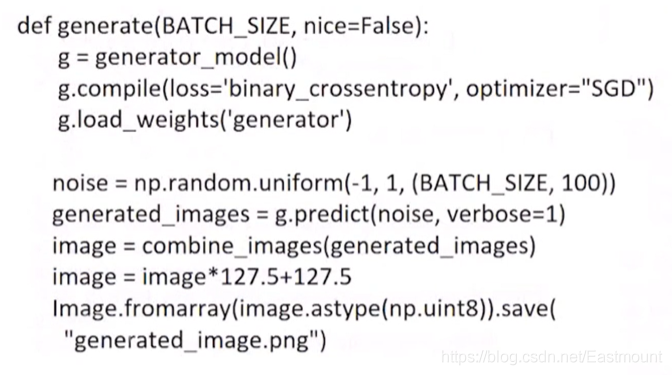# -*- coding: utf-8 -*-
"""
Created on 2021-03-19
@author: xiuzhang Eastmount CSDN

"""
from keras.models import Sequential
from keras.layers import Dense
from keras.layers import Reshape
from keras.layers.core import Activation
from keras.layers.normalization import BatchNormalization
from keras.layers.convolutional import UpSampling2D
from keras.layers.convolutional import Conv2D, MaxPooling2D
from keras.layers.core import Flatten
from keras.optimizers import SGD
from keras.datasets import mnist
import tensorflow as tf
import numpy as np
from PIL import Image
import argparse
import math
import os

## GPU處理 讀者如果是CPU註釋該部分程式碼即可
## 指定每個GPU程序中使用視訊記憶體的上限 0.9表示可以使用GPU 90%的資源進行訓練
os.environ["CUDA_DEVICES_ORDER"] = "PCI_BUS_IS"
os.environ["CUDA_VISIBLE_DEVICES"] = "0"
gpu_options = tf.GPUOptions(per_process_gpu_memory_fraction=0.8)
sess = tf.Session(config=tf.ConfigProto(gpu_options=gpu_options))

#----------------------------------------------------------------
#生成器
def generator_model():
model = Sequential()
return model

#----------------------------------------------------------------
#判別器
def discriminator_model():
model = Sequential()
Conv2D(64, (5, 5),
input_shape=(28, 28, 1))
)
return model

#----------------------------------------------------------------
#輔助函式 固定D調整G
def generator_containing_discriminator(g, d):
model = Sequential()
d.trainable = False
return model

#輔助函式 合併影象
def combine_images(generated_images):
num = generated_images.shape
width = int(math.sqrt(num))
height = int(math.ceil(float(num)/width))
shape = generated_images.shape[1:3]
image = np.zeros((height*shape, width*shape),
dtype=generated_images.dtype)
for index, img in enumerate(generated_images):
i = int(index/width)
j = index % width
image[i*shape:(i+1)*shape, j*shape:(j+1)*shape] = \
img[:, :, 0]
return image

#----------------------------------------------------------------
#訓練
def train(BATCH_SIZE):
(X_train, y_train), (X_test, y_test) = mnist.load_data()
X_train = (X_train.astype(np.float32) - 127.5)/127.5
X_train = X_train[:, :, :, None]
X_test = X_test[:, :, :, None]
#X_train = X_train.reshape((X_train.shape, 1) + X_train.shape[1:])
d = discriminator_model()
g = generator_model()
d_on_g = generator_containing_discriminator(g, d)
d_optim = SGD(lr=0.0005, momentum=0.9, nesterov=True)
g_optim = SGD(lr=0.0005, momentum=0.9, nesterov=True)
g.compile(loss='binary_crossentropy', optimizer="SGD")
d_on_g.compile(loss='binary_crossentropy', optimizer=g_optim)
d.trainable = True
d.compile(loss='binary_crossentropy', optimizer=d_optim)
for epoch in range(100):
print("Epoch is", epoch)
print("Number of batches", int(X_train.shape/BATCH_SIZE))
for index in range(int(X_train.shape/BATCH_SIZE)):
noise = np.random.uniform(-1, 1, size=(BATCH_SIZE, 100))
image_batch = X_train[index*BATCH_SIZE:(index+1)*BATCH_SIZE]
generated_images = g.predict(noise, verbose=0)
if index % 20 == 0:
image = combine_images(generated_images)
image = image*127.5+127.5
Image.fromarray(image.astype(np.uint8)).save(
str(epoch)+"_"+str(index)+".png")
X = np.concatenate((image_batch, generated_images))
y =  * BATCH_SIZE +  * BATCH_SIZE
d_loss = d.train_on_batch(X, y)
print("batch %d d_loss : %f" % (index, d_loss))
noise = np.random.uniform(-1, 1, (BATCH_SIZE, 100))
d.trainable = False
g_loss = d_on_g.train_on_batch(noise,  * BATCH_SIZE)
d.trainable = True
print("batch %d g_loss : %f" % (index, g_loss))
if index % 10 == 9:
g.save_weights('generator', True)
d.save_weights('discriminator', True)

#----------------------------------------------------------------
#GAN圖片生成
def generate(BATCH_SIZE, nice=False):
g = generator_model()
g.compile(loss='binary_crossentropy', optimizer="SGD")
if nice:
d = discriminator_model()
d.compile(loss='binary_crossentropy', optimizer="SGD")
noise = np.random.uniform(-1, 1, (BATCH_SIZE*20, 100))
generated_images = g.predict(noise, verbose=1)
d_pret = d.predict(generated_images, verbose=1)
index = np.arange(0, BATCH_SIZE*20)
index.resize((BATCH_SIZE*20, 1))
pre_with_index = list(np.append(d_pret, index, axis=1))
pre_with_index.sort(key=lambda x: x, reverse=True)
nice_images = np.zeros((BATCH_SIZE,) + generated_images.shape[1:3], dtype=np.float32)
nice_images = nice_images[:, :, :, None]
for i in range(BATCH_SIZE):
idx = int(pre_with_index[i])
nice_images[i, :, :, 0] = generated_images[idx, :, :, 0]
image = combine_images(nice_images)
else:
noise = np.random.uniform(-1, 1, (BATCH_SIZE, 100))
generated_images = g.predict(noise, verbose=1)
image = combine_images(generated_images)
image = image*127.5+127.5
Image.fromarray(image.astype(np.uint8)).save(
"generated_image.png")

#引數設定
def get_args():
parser = argparse.ArgumentParser()
parser.set_defaults(nice=False)
args = parser.parse_args()
return args

if __name__ == "__main__":
"""
args = get_args()
if args.mode == "train":
train(BATCH_SIZE=args.batch_size)
elif args.mode == "generate":
generate(BATCH_SIZE=args.batch_size, nice=args.nice)
"""
mode = "train"
if mode == "train":
train(BATCH_SIZE=128)
elif mode == "generate":
generate(BATCH_SIZE=128)

Training:
python dcgan.py --mode train --batch_size <batch_size>
python dcgan.py --mode train --path ~/images --batch_size 128

Image generation:
python dcgan.py --mode generate --batch_size <batch_size>
python dcgan.py --mode generate --batch_size <batch_size> --nice : top 5% images according to discriminator
python dcgan.py --mode generate --batch_size 128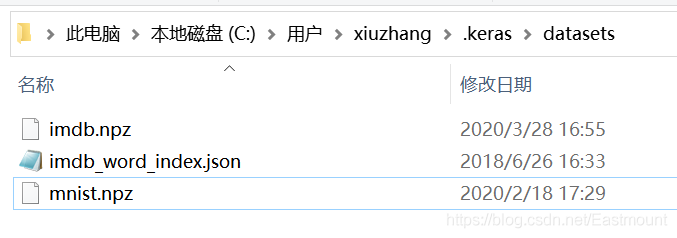Epoch is 0
Number of batches 468
batch 0 d_loss : 0.648902
batch 0 g_loss : 0.672132
batch 1 d_loss : 0.649307
....
batch 466 g_loss : 1.305099
batch 467 d_loss : 0.375284
batch 467 g_loss : 1.298173

Epoch is 1
Number of batches 468
batch 0 d_loss : 0.461435
batch 0 g_loss : 1.231795
batch 1 d_loss : 0.412679
....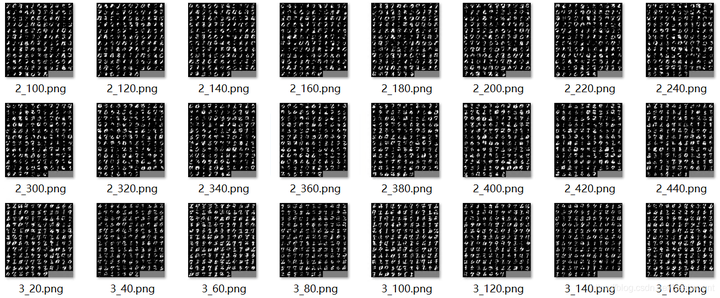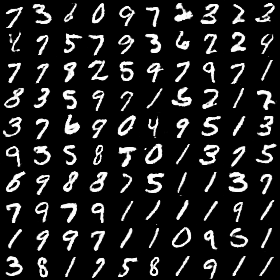(1) CGAN

CGAN出場。這裡簡單介紹下GAN和CGAN的區別：GAN只能判斷生成的東西是真的或假的，如果想指定生成影象如1、2、3呢？GAN會先生成100張影象，然後從中去挑選出1、2、3，這確實不方便。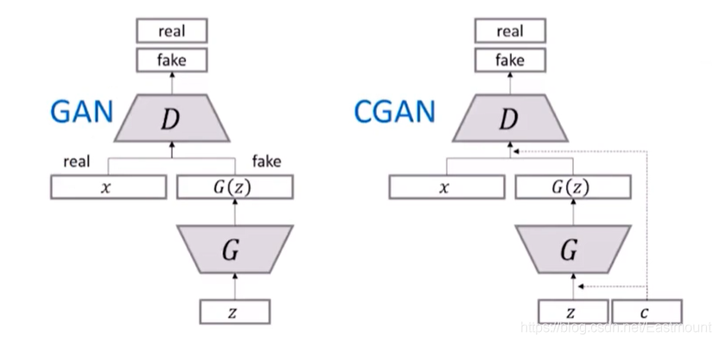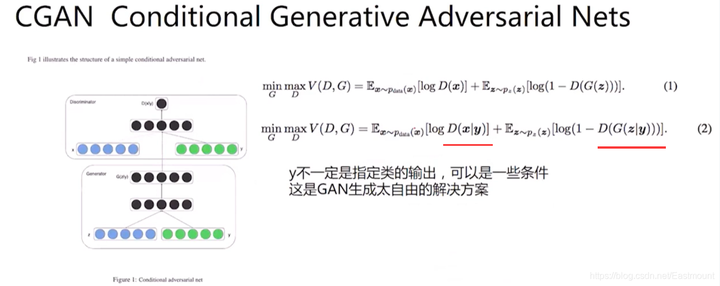(2) DCGAN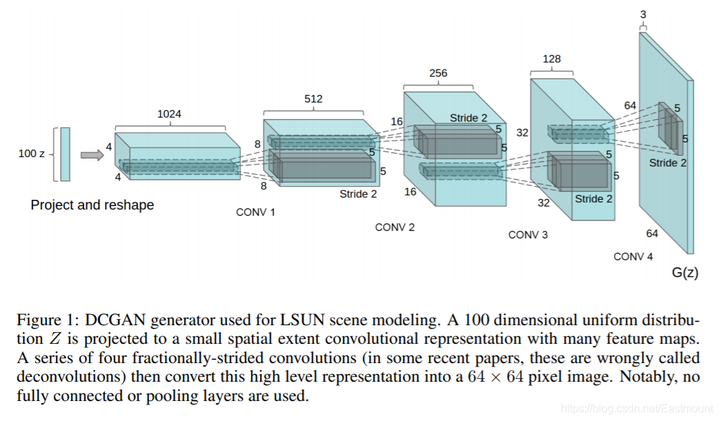DCGAN論文使用的tricks包括：

• 所有pooling都用strided convolutions代替，pooling的下采樣是損失資訊的，strided convolutions可以讓模型自己學習損失的資訊
• 生成器G和判別器D都要用BN層（解決過擬合）
• 把全連線層去掉，用全卷積層代替
• 生成器除了輸出層，啟用函式統一使用ReLU，輸出層用Tanh
• 判別器所有層的啟用函式統一都是LeakyReLU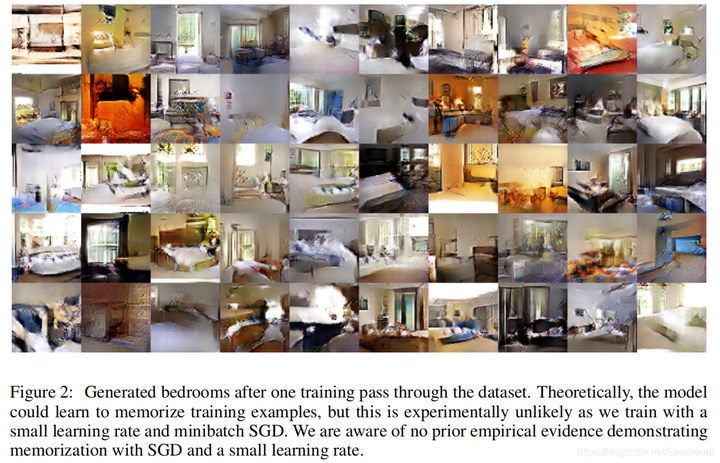(3) ACGAN

ACGAN（既能生成影象又能進行分類）
Conditional Image Synthesis with Auxiliary Classifier GANs，該判別器不僅要判斷是真（real）或假（fake），還要判斷其屬於哪一類。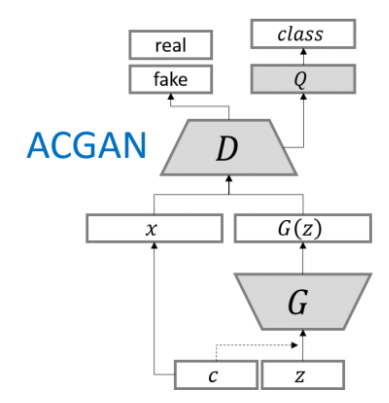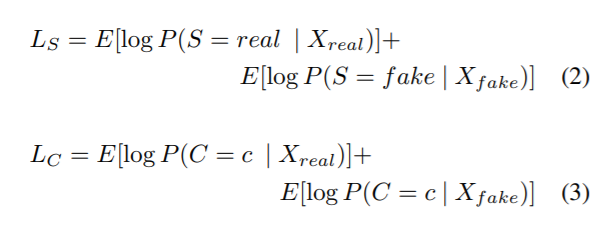(4) infoGAN

InfoGAN：Interpretable Representation Learning by Information Maximizing Generative Adversarial Networks。這個號稱是OpenAI在2016年的五大突破之一。

• D網路的輸入只有x，不加c
• Q網路和D網路共享同一個網路，只是到最後一層獨立輸出
• G(z)的輸出和條件c區別大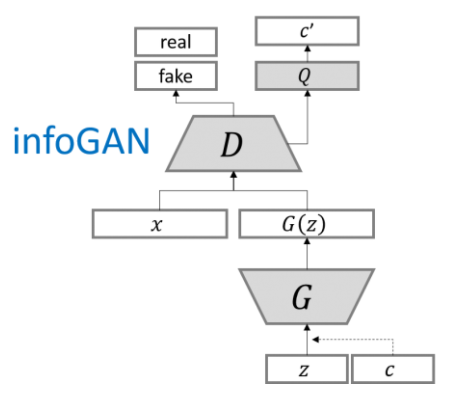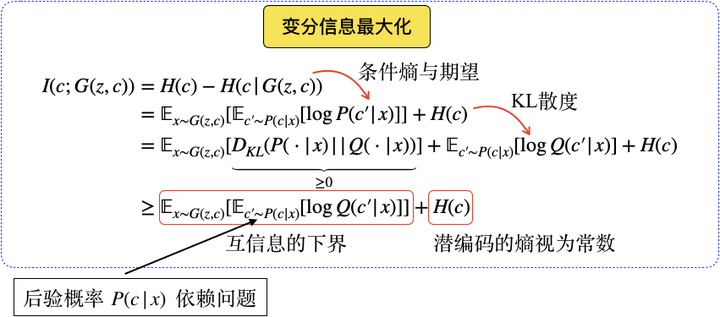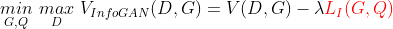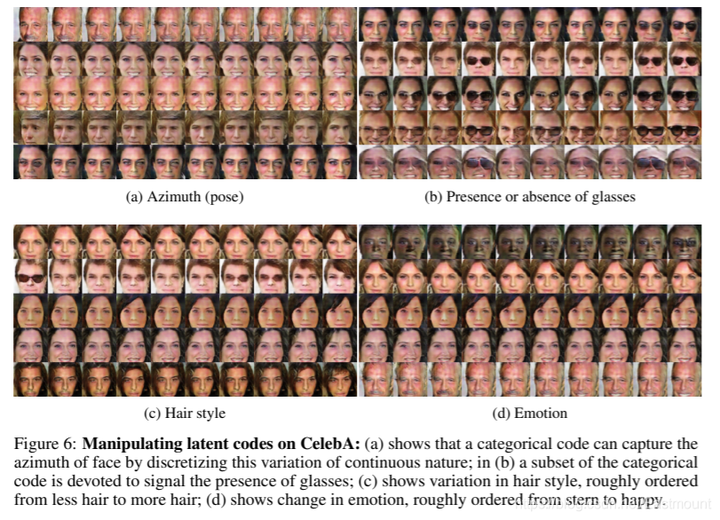(5) LAPGAN

• 首先用噪聲去生成一個小的圖片，解析度極低，我們對其拉伸。
• 拉伸之後，想辦法通過之前訓練好的GAN網路生成一個它的殘差。
• 殘差和拉伸圖相加就生成一張更大的圖片，以此類推，拉普拉斯生成一張大圖。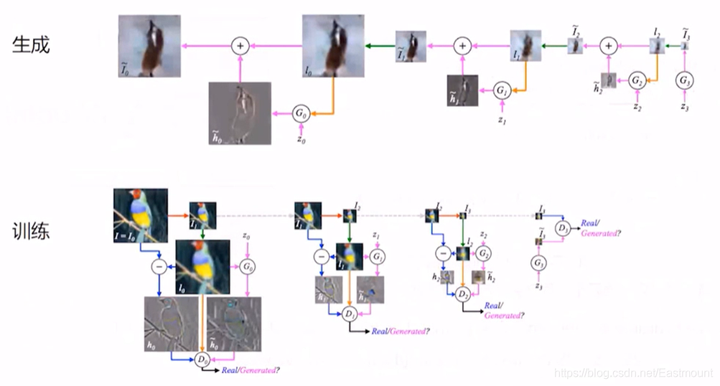(6) EBGAN

• 《Energy-based Generative Adversarial Network》Junbo Zhao, arXiv:1609.03126v2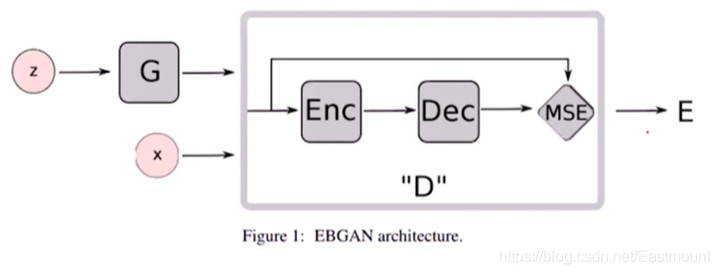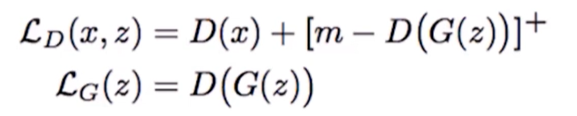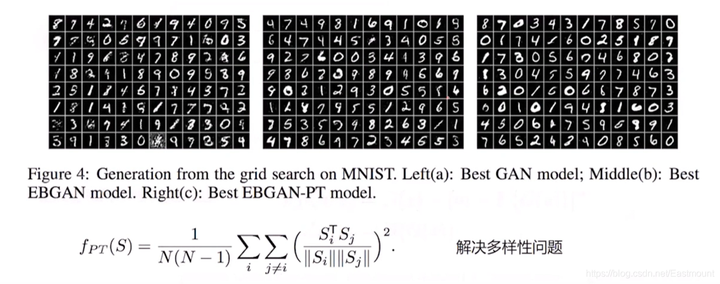4.GAN改進策略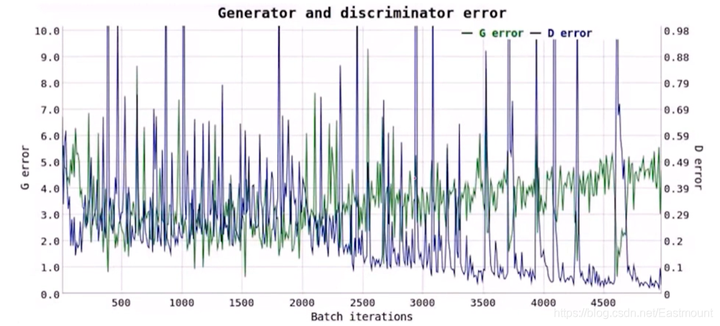GAN需要重視：穩定（訓練不奔）、多樣性（各種樣本）、清晰度（質量好），現在很多工作也是解決這三個問題。

• G、D迭代的方式能達到全域性最優解嗎？大部分情況是區域性最優解。
• 不一定收斂，學習率不能高，G、D要共同成長，不能其中一個成長的過快
– 判別器訓練得太好，生成器梯度消失，生成器loss降不下去
– 判別器訓練得不好，生成器梯度不準，四處亂跑
• 奔潰的問題，通俗說G找到D的漏洞，每次都生成一樣的騙D
• 無需預先建模，模型過於自由，不可控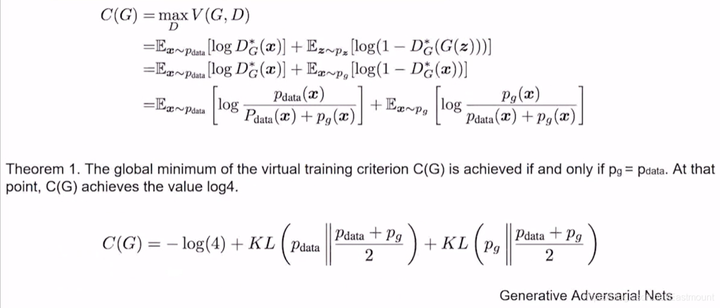• “生成器沒能生成真實的樣本” 懲罰小
• “生成器生成不真實的樣本” 懲罰大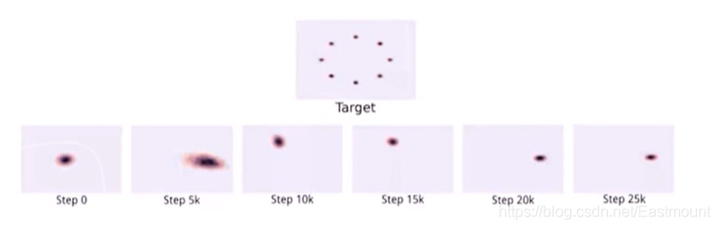WGAN（Wasserstein GAN）在2017年被提出，也算是GAN中里程碑式的論文，它從原理上解決了GAN的問題。具體思路為：

• 判別器最後一層去掉sigmoid
• 生成器和判別器的loss不取log
• 每次更新判別器的引數之後把它們的絕對值截斷到不超過一個固定的常數c
• 用Wasserstein距離代替KL散度，訓練網路穩定性大大增強，不用拘泥DCGAN的那些策略（tricks）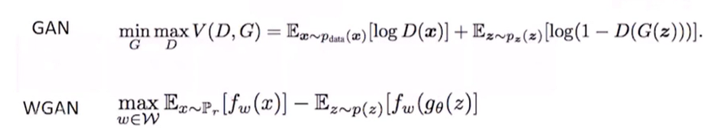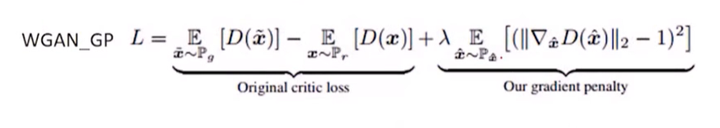• https://arxiv.org/pdf/1705.07215.pdf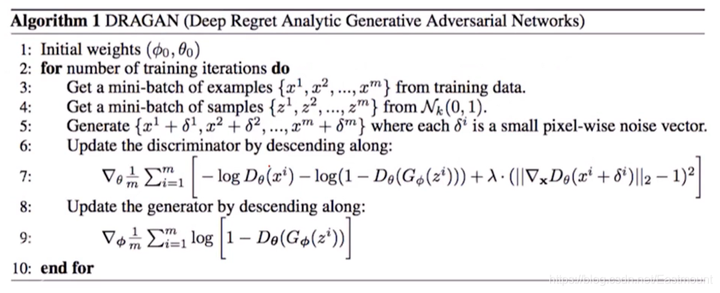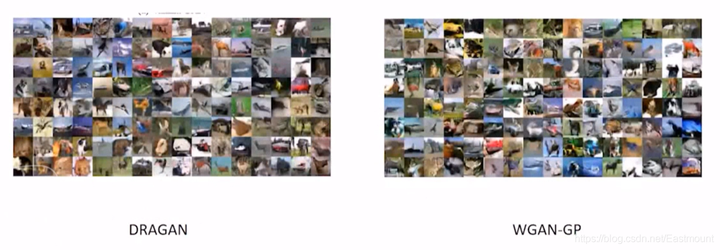《Google Brain: Are GANs Created Equal? A Large-Scale Study》 這篇論文詳細對比了各GAN模型點心LOSS優化變種。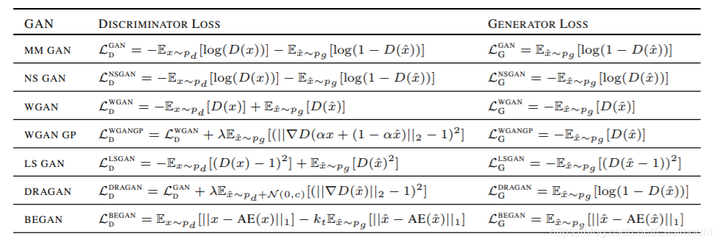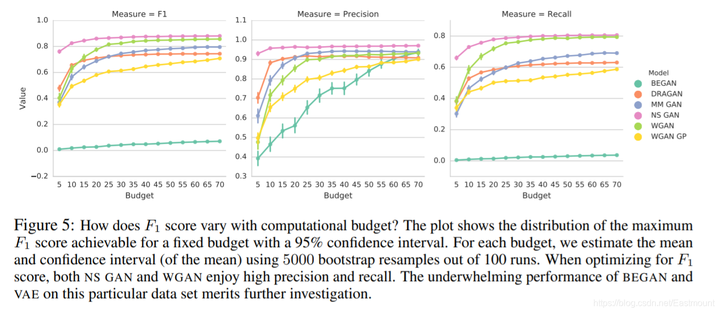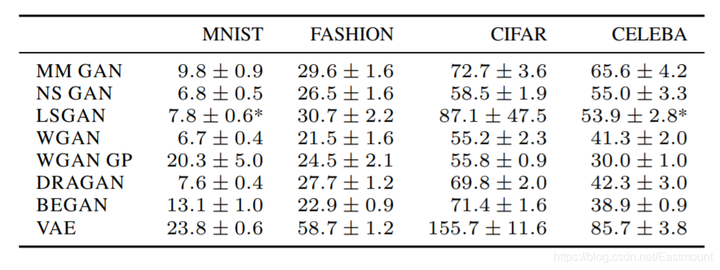四.總結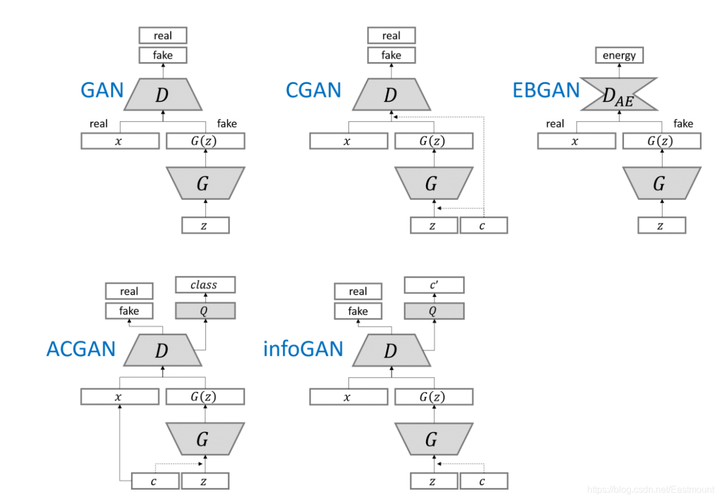• https://github.com/eastmountyxz/AI-for-Keras
• https://github.com/eastmountyxz/AI-for-TensorFlow

參考文獻：

• https://www.bilibili.com/video/BV1ht411c79k
• https://arxiv.org/abs/1406.2661
• https://www.cntofu.com/book/85/dl/gan/gan.md
• https://github.com/hindupuravinash/the-gan-zoo
• https://arxiv.org/pdf/1701.00160.pdf
• https://zhuanlan.zhihu.com/p/76520991
• http://cn.arxiv.org/pdf/1711.09020.pdf
• https://www.sohu.com/a/121189842_465975
• https://www.jianshu.com/p/88bb976ccbd9
• https://zhuanlan.zhihu.com/p/23270674
• ttps://http://blog.csdn.net/weixin_40170902/article/details/80092628
• https://www.jiqizhixin.com/articles/2016-11-21-4
• https://github.com/jacobgil/keras-dcgan/blob/master/dcgan.py
• https://arxiv.org/abs/1511.06434
• https://arxiv.org/pdf/1511.06434.pdf
• https://blog.csdn.net/weixin_41697507/article/details/87900133
• https://zhuanlan.zhihu.com/p/91592775
• https://liuxiaofei.com.cn/blog/acgan與cgan的區別/
• https://arxiv.org/abs/1606.03657
• https://blog.csdn.net/sdnuwjw/article/details/83614977
• 《Energy-based Generative Adversarial Network》Junbo Zhao, arXiv:1609.03126v2
• https://www.jiqizhixin.com/articles/2017-03-27-4
• https://zhuanlan.zhihu.com/p/25071913
• https://arxiv.org/pdf/1705.07215.pdf
• https://arxiv.org/pdf/1706.08500.pdf
• https://arxiv.org/pdf/1711.10337.pdf
• https://www.zhihu.com/question/263383926